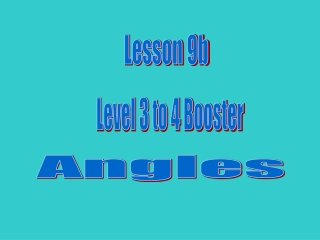# Lesson 9b - PowerPoint PPT PresentationDownload PresentationLesson 9b

Presentation Description
Download Presentation## Lesson 9b

- - - - - - - - - - - - - - - - - - - - - - - - - - - E N D - - - - - - - - - - - - - - - - - - - - - - - - - - -
##### Presentation Transcript

1. Lesson 9b Level 3 to 4 Booster Angles

2. Objective: Know the sum of angles at a point, on a straight line and in a triangle. Vocabulary: straight angle right angle degrees

3. 900 900 900 900 900

4. right angle

5. 400 500 ?0

6. 550 300 720 630 530

7. 550 300 180 600 720 350 630 510 390 270

8. 1800 straight angle

9. 1800 1800 1800 1800

10. 500 ?0 1300

11. 550 600 1150 1120 350

12. 550 600 1150 1200 1250 650 1450 680 1120 350

13. 3600

14. 550 3050

15. 550 900 + 550 = 1450 3600 – 1450 = 2150 2150

16. 850 1150 2750 2450 850 1450 1900 500 750 850

17. ruler ruler ruler ruler ruler ruler ruler ruler ruler ruler ruler ruler ruler ruler ruler ruler ruler

18. The sum of the angles in a triangle is 1800

19. The sum of the angles in a triangle is 1800

20. The sum of the angles in a triangle is 1800 850 + 500 = 1350 1800 – 1350 = 450 ? = 450 850 500 ?

21. The sum of the angles in a triangle is 1800 300 + 1100 = 1400 1800 – 1400 = 400 ? = 400 1100 ? 300

22. The sum of the angles in a triangle is 1800 550 + 520 = 1070 1800 – 1070 = 730 ? = 730 520 ? 550

23. The sum of the angles in a triangle is 1800 750 + 600 = 1350 1800 – 1350 = 450 ? = 450 ? 600 750

24. Thank you for your attention